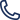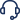530-820-3390 530-820-3390Support

## Sequencenumber function

This function is used to generate a sequence of number as required by the user. This function takes in three parameters and third one is optional. If user does not provide third parameter it will take the start value as zero.

First parameter: Reset parameter is a Boolean and will take true or false and by default it should be false.
Second parameter: Number of digits is the count of digit user wants.
Third parameter: Start value is starting value of the sequence.

Syntax: \${__sequencenumber(reset parameter,number of digits, start value)}

Edit parameter section and click on Form, select functions from the dropdown and select SEQUENCENUMBER function. Select reset value as true or false (If true is selected every time this function is called it will start from the given value and if false is selected it will continue adding itself by one from the starting sequence number ), provide number of digits and start number of the sequence.

After completing this step, it will look as below:

Sequencenumber generated will be stored in a variable defined in Element key.

Example: \${__sequencenumber(false,5,100)}
Output: Value will be “00101”
Now to get the next sequence number of this pattern user need to give the same function with only 2 parameters as shown below:
Example: \${__sequencenumber(false,5)}
Output: 00102

Example: \${__sequencenumber(false,5)}
Output: 00103

Example: \${__sequencenumber(false,5)}
Output: 00104

Similarly user can generate n number of sequence by calling the sequence number function again and again.

Note: After using this function in one test case if you want to use this function again in another test case again from scratch then it should be first reset.
Syntax: \${__sequencenumber(true,5)}

You can get the random number of any number of digits ranging between 5 to 16 i.e. second parameter in this function(number of digits) can take vales from 5 to 16 for desired output.